Display case

Place a glass shelf at the height of 1m from the bottom of the display case in the cabinet. How long platter will we place at this height?

The display case is a rectangular triangle with 2 m and 2.5 m legs.

Result

x =  1.2 m

Solution:Leave us a comment of example and its solution (i.e. if it is still somewhat unclear...):Be the first to comment!To solve this verbal math problem are needed these knowledge from mathematics:

Do you want to convert length units? See also our trigonometric triangle calculator.

Next similar examples:

1. RunnersFor three runners (on the first to third place) is prepared 30 chocolate that they be distributed in the ratio of 3 : 2 : 1 How much chocolate will get everyone?
2. Photo enlarge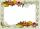Mark wants to enlarge a 4-inch by 6-inch photo so that it has a height of 15inches. Use a ratio table to determine the new width of the photo.
3. MedianThe median of the triangle LMN is away from vertex N 84 cm. Calculate the length of the median, which start at N.
4. Guppies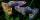Audrey has some guppies in a fish tank. The ration of the oranges guppies to silver guppies is 3:5. She has 12y oranges guppies. Write the number of silver guppies she has in terms of y
5. Calories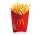A large order of McDonald’s french fries has 500 calories. Of this total, 220 calories are from fat. Find the ratio of the calories from fat to total calories in a large order of McDonald’s french fries.
6. String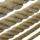Divide the string length 800 cm in ratio 1: 5
7. Snowman 3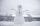During the last winter carnival, the local college students built a 30- foot snowman out of 109 tons of snow. How much snow will be needed to build a 36- foot snowman this year?
8. BuildingAt the building, we divided 240 boards into two piles in a 5: 3 ratio. How many were fewer boards in the lower pile?
9. Good swimmerGood swimmer swims 23 m distance with ten shots. With how many shots he swim to an island located 81 m if still swims at the same speed?
10. Two anglesThe triangles ABC and A'B'C 'are similar. In the ABC triangle, the two angles are 25° and 65°. Explain why in the triangle A'B'C 'is the sum of two angles of 90 degrees.
11. Moneys in triple ratioMilan, John and Lili have a total 344 euros. Their amounts are in the ratio 1:2:5. Determine how much each of them has?
12. AgesJohn, Teresa, Daniel and Paul have summary 56 years. Their ages are in a ratio of 1:2:5:6. Determine how many years have each of them.
13. Divide moneyDivide 1200 USD at a ratio of 1:2:3:4:5:6:9:10
14. PeppersIn the box are yellow (a), green (b) and red (c) peppers. Their amount is in a ratio 2:4:1 . Most are yellow peppers and green the least. Calculate the number of peppers each type if the total number of peppers is 70.
15. RatiosReduce the numbers: 50 in a 1:2 ratio 111 at a ratio of 2:3 70 at 10:50 560 at a ratio of 3:8
16. Pills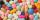If it takes 20 minutes to run a batch of 100 pills how many minutes would it take to run a batch of 50 pills
17. Tailor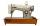Tailor bought 2 3/4 meters of textile and paid 638 CZK. Determine the price per 1 m of the textile.